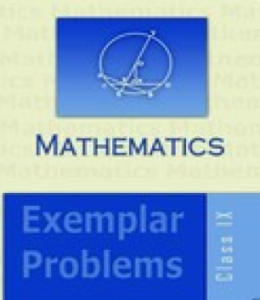# NCERT EXEMPLAR BOOK CLASS 9 Maths

## CBSE NCERT EXEMPLAR BOOK CLASS 9 Maths

Mathematics can be easier if the students are strong with the fundamentals. Thus, NCERT Exemplar Book Class 9 Maths comes to the rescue. Firstly, the practice book will help the students progress in the mathematics subject. Secondly, The conceptual sums can be easily decoded if the students spend enough in time solving the NCERT Exemplar Book Class 9 Maths. The questions are varied: from multiple-choice to twisted. The conceptual sums of NCERT Exemplar Books help the student increase his or her analytical skills.

NCERT Exemplar Maths Book Class 9 will help the students to be thorough with formulas and theories. In fact, the books will help them to solve the questions in more than one way. More importantly, students will also get to know the shortcuts and tips when it comes to solving twisted problems. Each chapter is described in details. Therefore, it provides an opportunity for the students to have more focus on the application of concepts, rather than learning the concepts.

In total, there are 14 chapters in NCERT Exemplar Book Class 9 Maths. It covers the basics and fundamentals of mathematics on all topics. In addition, it has added information on a higher level for various competitive examinations, such as NTSE, NIMO, etc. NCERT Exemplar Book Class 9 Maths prepare the students for competitive exams like JEE Main, JEE Advanced, NEET etc because they are specifically designed for entrance exams. In conclusion, the NCERT Exemplar Book Class 9 Maths will make everyone fall in love with Mathematics.